Home > ACC7 > Chapter cc38 > Lesson cc38.3.1 > Problem8-134

8-134.
1. Compute each product or quotient. Convert the final answer to scientific notation if necessary. Homework Help ✎

1. (2 × 102)(3.2 × 10−5)

2. (4 × 102)(2.5 × 10−3)

3.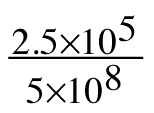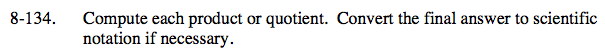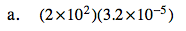What is 2 · 3.2?

What is 102 · 10−5?

6.4 × 10−3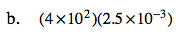See part (a).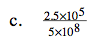$\text{What is }\frac{2.5}{5}?$

$\text{What is }\frac{10^{5}}{10^{8}}?$

0.5 · 10−3
Is this in scientific notation?